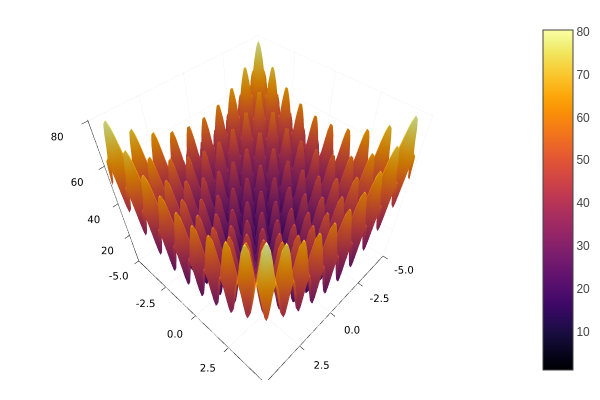# Metaheuristics - an Intuitive Package for Global Optimization

High-performance algorithms for optimization coded purely in a high-performance language.

## Introduction

Optimization is one of the most common tasks in the scientific and industrial field but real-world problems require high-performance algorithms to optimize non-differentiable, non-convex, discontinuous functions. Different metaheuristics algorithms have been proposed to solve optimization problems but without strong assumptions about the objective function.

This package implements state-of-the-art metaheuristics algorithms for global optimization. The package aims to provide easy-to-use (and fast) metaheuristics for numerical global optimization.

## Installation

Open the Julia (Julia 1.1 or Later) REPL and press ] to open the Pkg prompt. To add this package, use the add command:

pkg> add Metaheuristics

Or, equivalently, via the Pkg API:

julia> import Pkg; Pkg.add("Metaheuristics")

## Quick Start

Assume you want to solve the following minimization problem.Minimize:

$$$f(x) = 10D + \sum_{i=1}^{D} x_i^2 - 10\cos(2\pi x_i)$$$

where $x\in[-5, 5]^{D}$, i.e., $-5 \leq x_i \leq 5$ for $i=1,\ldots,D$. $D$ is the dimension number, assume $D=10$.

### Solution

Firstly, import the Metaheuristics package:

using Metaheuristics

Code the objective function:

f(x) = 10length(x) + sum( x.^2 - 10cos.(2π*x)  )

Instantiate the bounds:

D = 10
bounds = boxconstraints(lb = -5ones(D), ub = 5ones(D))

Also, bounds can be a $2\times 10$ Matrix where the first row corresponds to the lower bounds whilst the second row corresponds to the upper bounds.

Approximate the optimum using the function optimize.

result = optimize(f, bounds)
Optimization Result
===================
Iteration:       883
Minimum:         0.994959
Minimizer:       [-4.48345e-09, -9.30599e-10, 3.25831e-10, …, -0.994959]
Function calls:  61810
Total time:      0.3720 s
Stop reason:     Due to Convergence Termination criterion.


Optimize returns a State datatype which contains some information about the approximation. For instance, you may use mainly two functions to obtain such an approximation.

minimum(result)
0.9949590570932969
minimizer(result)
10-element Vector{Float64}:
-4.4834466353035e-9
-9.305989054639169e-10
3.258305882527824e-10
1.2886522823709618e-9
-2.8635734772523426e-9
-5.632279074993702e-10
-3.368283468758625e-9
-1.0454825577878258e-9
-4.519765429079066e-9
-0.9949586384993971

## How to cite?

Please cite the package using the bibtex entry

@article{metaheuristics2022,
doi = {10.21105/joss.04723},
url = {https://doi.org/10.21105/joss.04723},
year = {2022},
publisher = {The Open Journal},
volume = {7},
number = {78},
pages = {4723},
author = {Jesús-Adolfo Mejía-de-Dios and Efrén Mezura-Montes},
title = {Metaheuristics: A Julia Package for Single- and Multi-Objective Optimization},
journal = {Journal of Open Source Software} }

or the citation string

Mejía-de-Dios et al., (2022). Metaheuristics: A Julia Package for Single- and Multi-Objective Optimization. Journal of Open Source Software, 7(78), 4723, https://doi.org/10.21105/joss.04723

in your scientific paper if you use Metaheristics.jl.

## Acknowledgments

Jesús Mejía acknowledges support from the Mexican Council for Science and Technology (CONACyT) through a scholarship to pursue graduate studies at the University of Veracruz, MEXICO. This allowed the development of Metaheuristics.jl from August 2018 to July 2022.